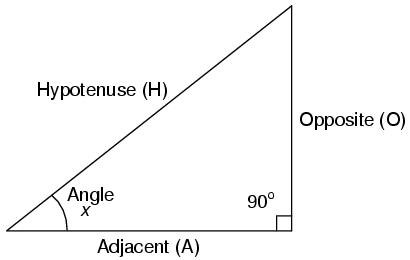# Set 3D view so 2D texture is exact size?

Hi,
Is it possible to setup a 3D projection so that a texture will be exactly magnified/minified to a given size? For instance, say I have a large chequer board texture with the squares 32x32 pixels in size. How do I set up the projection so that the squares are exactly 16x16? Hopefully this makes sense.

I guess the two values I need to manipulate are the Z distance and the field of view.

``````Thanks,
``````

Stuart.

Yes, it sounds like you need orthographic projection, since any perspective field-of-view will introduce sampling differences in screen coordinates.

Let’s say your checkerboard is 2x2, which gives total pixel dimensions of 64x64. You then know that the area on screen you want to draw to is (216)x(216) = 32x32. A simple way of achieving this is to use an orthographic projection and draw the textured quad in screen coordinates with the specified dimensions.

Apologies if this post is unclear, but I am sure you can find plenty of references on how to draw a quad in screen coordinates.

Sounds like you want this to be an image in the background of a scene. If so, I’d resize the image to exactly the size you need before bringing it into your OpenGL application. Use Photoshop or something similar to do this. Then I’d use glDrawPixels with an orthographic projection to position it on the screen. I’ve posted a small, self-contained, OpenGL program that loads images and displays them as textures or simply as images (using glDrawPixels). It’s at:

``  [http://www.mfwweb.com/OpenGL/Loading_Textures/](http://www.mfwweb.com/OpenGL/Loading_Textures/)``

Sorry, should have been clearer. I don’t want an orthographic projection I want a perspective projection, but position the texture (on a quad) so that it is exactly a given size.

For example I have a texture with 32x32 tiles drawn on it. With a FOV of 33 if I position the camera 648 away from the quad then the tiles on the texture are 64x64. If the camera is 648*2 away then the tiles are 32x32 and the quad looks the same as a 2d projection with a 1 to 1 pixel mapping. However I found the value just by trial and error and doing screen captures. I’d like to do the maths instead.

So I have a texture drawn on a quad viewed “flat on” (parallel to the screen) with a 3d projection and I want to know how to calculate the Z distance so that the quad will be a given size on the screen. I guess an alternative would be to use a 2D view and then glScale.

Hopefully that is a little clearer.

Let’s assume:
[ol][li]symmetric perspective projection, and[*]the quad you’re placing the texture on is perpendicular to the eye-space Z axis (i.e. parallel to the screen)[/ol]This is just a little trig problem. [/li]where:
eyepoint is at the vertex AH
angle = FOV / 2
A = distance to object
O = half the width of frustum at that distance

so:

O = tan(FOV/2) * A

where:

O*2 = w = width of view frustum at distance A.

So:

w = 2 * tan(FOV/2) * distance

And of course, that’s the width of the whole frustum at that distance. If you want to know the width of a pixel at that distance, then it’s approximately “w/res”.

With this you can figure how big to draw the quad you are drawing your texture to make it (or the texels in it) subtend the desired portion of the FOV at the appropriate distance.

Cheers that works perfectly.